# Filter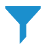Filter

With filter widgets, you can control which data is displayed in the visualizations on a storyboard page. By applying the filters, you reduce the amount of data that you need to analyze. This helps you focus only on the data of your interest.

## When to use

Use a filter widget to reduce the amount of data that you want to display in your visualizations. For example, if a visualization presents your company's sales per month, you can apply a filter to view only the sales for a specific product line.

You can use the following filter types:

• Dropdown – Select one value or all the values from the dropdown list. The drop-down list shows the records from the column that is used for filtering.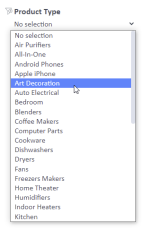This filter type is available if you select a column that contains dimensions.

• Single select – Select one option from the list. The list shows the records from the column that is used for filtering.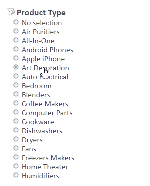This filter type is available if you select a column that contains dimensions.

• Multi select – Select several values from the list. The list shows the records from the column that is used for filtering.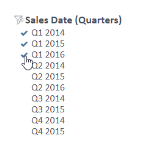This filter type is available if you select a column that contains dimensions.

• Expression – Enter a mathematical expression that you want to use for filtering the data in a non-aggregated status. For example, if you use the Sales Cost column for filtering and enter the expression >100, the filtered visualization shows only the data items for which the sales cost is higher than 100.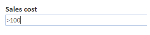You can use the following symbols.

SymbolUse this item to
=

Show only the data items whose value is equal to the specified value. For example, use "=100" to show only the data whose value is equal to 100.

>

Show only the data items whose value is greater than the specified value. For example, use ">100" to show only the data whose value is greater than 100.

>=

Show only the data items whose value is greater than or equal to the specified value. For example, use ">=100" to show only the data whose value is greater than or equal to 100.

<Show only the data items whose value is less than the specified value. For example, use "<100" to show only the data whose value is less than 100.
<=

Show only the data items whose value is less than or equal to the specified value. For example, use "<=100" to show only the data whose value is less than or equal to 100.

><

<>

Show a range of numeric values. For example, use ">100<500" to show only the data whose value is more than 100 and less than 500, or "<500>100" to show only the data whose value is less than 500 and more than 100.

>= <=

<= >=

Show a range of numeric values. For example, use ">=100<=500" to show only the data whose value is greater than or equal to 100 and less than or equal to 500, or "<=500>=100" to show only the data whose value is less than or equal to 500 and greater than or equal to 100.

This filter type is available if you select a column that contains measures.

You can apply filters to the following visualization types.

## Use case

View the following examples for using filter widgets.

Tip: Depending on your needs, you can choose to have multiple filter widgets instead of one filter widget with several filters on a storyboard.

## References

For details on how to customize your widget, see Visualization settings (appearance tab).

For a whole list of visualizations, see the following topics: